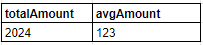# MySQL: Write a query to retrieve the total amount received from all orders as well as the average amount and round answers to nearest integer

, 01/06/2022 - 20:20

### Rounded Total and Average Order Amounts

Description: Write a query to retrieve the total amount received from all orders, as well as the average amount and round the answers to the nearest integer.

The ROUND() function rounds a number to a specified number of decimal places.

```ROUND(number, decimals)
```

number: required

decimals: optional

Note:

Table name: orderdetails

use priceEach * quantityOrdered to find the amount.

Sample OutputHi,

You can use the following query:

``````select (price_each * quantity_ordered) as totalAmount,
avg(price_each * quantity_ordered) as avgAmount,
round(price_each * quantity_ordered) as totalAmount_round,
round(avg(price_each * quantity_ordered)) as avgAmount_round
from order_details;``````

Hi ,
This is not giving the expected result. please check.

The correct query is given below :

``````select round(sum(priceEach * quantityOrdered)) as totalAmount,
round(avg(priceEach * quantityOrdered)) as avgAmount
from orderdetails;``````